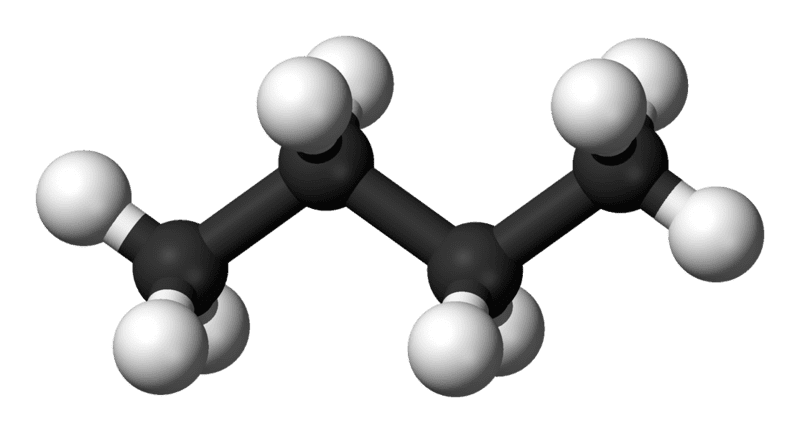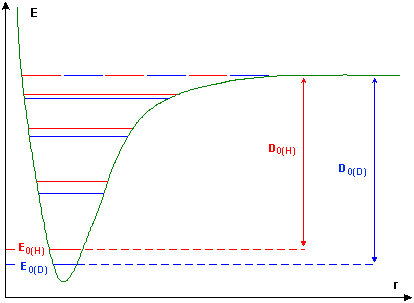# Gaussian - What exactly does the second derivative of the PES represent

mycotheology
I posted this in two forums because the question might be too chemistry related for the people in the calculus forum.

I'm a visual thinker so I struggle a bit to get my head around calculus concepts. So as an example, heres a potential energy surface:

lets say this represents the structure of a simple molecule like n-propane:the molecule in the picture is the most stable conformation so the global minimum (the big pit) on the PES represents that conformation. Then the local minimum (the little bit) represents the staggered conformation. If I rotate or stretch the bonds in any other way, then it becomes less stable so the molecules energy will no longer be represented by a minimum on the PES.

I can see how the first derivative will help you locate the minima because the slope will be 0 there. The first derivative of this potential energy surface is called the "force" which makes sense to me because the y axis represents the potential energy so when the molecule is in a stable conformation, the force which would ordinarily push the molecule into a stable conformation (if it was in an unstable one) is 0. What I'm trying to get my head around now is what the 2nd derivative represents. They call it the "force constant" and I know that in computational chemistry, they'll calculate the "force constant matrix" for a molecule. I'm trying to figure out what that means.

Last edited by a moderator:

## Answers and Replies

Einstein Mcfly
Well, what do first and second derivatives tell you about general functions?

mycotheology
The first derivative of a function e.g. f(x) = x3 - 27x tells you its slope at a particular point on the graph, so setting the first derivative to 0 e.g. f'(x) = 3x2 - 27 = 0 then solving for x will tell you where there are stationary points so in this case there are stationary points at 3 and -3. Its been a couple of years since I did calculus, I just remembered that the 2nd derivative is used to tell you whether the stationary point is a maximum or minimum. So if I plug the stationary points into the 2nd derivative, f''(3) = 6x = 18, f''(3) = 6x = -18 I know that 3 is a minimum and -3 is a maximum. I read that the presence of negative vibrations in a freq scan tells you that the structure is not optimised properly, and that it rests on a local minimum rather than the global minimum. More specifically they mentioned saddle points. I don't understand how IR frequencies relate to structure optimisation but I'm guessing the negative value has something to do with the fact that a saddle point is a maximum if you approach it in a particular direction but I don't know how they could indicate the presence of a regular local minimum.

I have a book on Gaussian but it doesn't really explain the theory in-depth behind how Gaussian does what it does. Like the Gaussian 09 manual, all it says about frequency scans is "they use the second derivative of the energy with respect to nuclear position to calculate the frequencies". Those words aren't enough for me to gain an understanding unfortunately. In a molecular vibration (lets say a stretching vibration) involving 2 atoms of the molecule, the bond length increases and decreases, I'm guessing this somehow shows on the potential energy surface. Do you know of a tutorial or book that gives detailed, in-depth explanations of the theory behind all this? You seem to have a deep understanding of all this, did you learn from a book? Your explanation about what a stability scan actually does with respect to spin multiplicities, thats the kinda thing I'm looking for, explanations that give me an understanding of the theory behind all this. A proper explanation like that is nowhere to be found on the internet it seems.

Last edited:
JohnRC
Oh dear, Myco! The molecule you are dealing with up in the first post is n-butane, not propane, but that is a fairly minor problem!

Let me give you a very straight answer to what the second derivative represents -- it is, in essence, a curvature. If you imagine a tangent plane to a many dimensional potential surface, then a positive second derivative means that the potential surface curls upward away from the plane, while a negative second derivative means that it curls downward. It is not, by the way, a *curvature* according to the strict mathematical definition, but I think a fellow visual thinker will recognise what I mean by using the word.

Increasing *curvature* is something you would associate with morphing from a straight line into an ever tightening circle; increasing second derivative would alternatively be associated with fixing the middle, and morphing with an upward stretch at the edges.

I cannot recommend a textbook, because I do not know one that treats this subject at all well, and because I seldom use textbooks (having long ago found that they are not written with visual thinkers in mind). I can recommend looking at contour maps of topography and thinking about the associated landforms.

In one dimension, a stationary point, where the gradient becomes zero, must be a maximum or a minimum. The second derivative will specify which -- positive for a minimum; negative for a maximum. In two dimensions, there are three different types of place where the terrain is locally level: the bottom of a sinkhole is a minimum. There are two second derivatives, and both are positive. The top of a hill is a maximum; in this case both second derivatives are negative. There is a third type of locally flat terrain that has no 1-dimensional analogue: a saddle point has one positive and one negative second derivative. It represents both the lowest point on a ridge that connects two hilltops, and the lowest pass from one valley to another -- or the highest point on a passage from one valley to another.

When you use Gaussian for a medium sized molecule, the potential surface you are working with is many-dimensional. It will have many stationary points, where there is locally level ground. There are only three types that are of interest.

• A local minimum, where all second derivatives are positive, represents a stable structure.
• A saddle point, where there is only one negative second derivative, represents a transition state, the highest point on the lowest path connecting two different minima. This plays an important part in calculations connected with chemical reactions rather than chemical structures.
• A "false saddle" where there are two, or possibly more negative second derivatives. Many years ago I did a lot of work on a reaction
CH2NH2+ → HCNH+ + H2
Every species in that reaction is planar, so I thought to reduce the dimensionality of the problem by leaving out the z co-ordinate of each atom. It turned out to be a bad move, because there is a lower path from reactants to products if the system takes a bit of a twist near the false saddle that I found to pass through one of the two symmetrically equivalent genuine saddle points with non-planar arrangements of the atoms. My false saddle had a negative second derivative for one of the out-of-plane co-ordinates that I had neglected, as well as the one "reaction co-ordinate" negative second derivative that I knew about.

mycotheology
Thanks a lot JohnRC! I'm starting to get my head around all this now. Good to see an explanation from a fellow visual thinker. I know what you mean about textbooks not being written for visual thinkers, I have the same problem with lectures and the majority of tutorials on the internet. I find that the big, thick textbooks usually provide pictures though which is an enormous help. Luckily in this day and age, you can find all kinds of pictures and animations online, sometimes I find 3D animations on youtube which allow me to learn a concept in minutes, that woulda taken me God knows how long if I was reading or listening to words describing it.

After reading you're post, I understand the concepts of curvature and why the number of second deratives there are for depends on the number of dimensions involved. What I really want to understand now is how IR vibrations come into all this. In a freq scan, I know that Gaussian calculates a negative vibrational frequency if the structure is a saddle point and numerous negative frequencies for higher order saddle points so I can see a clear correlation between the second derivative and the calculated frequencies but I'm trying to visualise how they are actually related. Lets use carbon monoxide as an example. Its a linear diatomic molecule so it has only a single stretching vibration (which is IR active if I'm not mistaken). How does Gaussian use the PES to find this vibration? If I'm not mistaken, a diatomic molecule has a 2 dimensional PES because it can only stretch and contract. I'm guessing its PES looks like an anharomic oscillator:the minimum of this PES only has 1 second derivative. I'm starting to see how this pit corresponds to molecular vibration but how does Gaussian know where the normal modes of vibration are located in this pit?

Last edited: